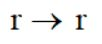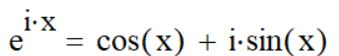### Molecular Spectroscopy, Part 1: Rotating Systems and Quantum Mechanics

As I mentioned in a previous article, I was waiting to discuss Spectroscopy until the end of the semester, and since such time has finally come to pass, it's time to offer another segment to my analytical techniques series covered here on my blog. I will however be taking these next few pieces slow due to the fact that these next sets of phenomena, rotational and vibrational spectroscopy, require being described through quantum mechanics in order to be fully understood, and the phenomena of quantum mechanics is difficult to understand, to say the least. I'll try my best to offer an understanding that can at least get us closer to contemplating the "weirdness" that arises when dealing within the realm of the small.

I want to start with rotational spectroscopy over its counterpart in vibrational spectroscopy due to the "relative" simplicity regarding the introduction of quantization, which is probably the most non-intuitive aspect of the natural world. Quantization is a phenomena that was first observed, and quantum mechanics was the mechanism to which it was explained (I covered this a bit in my term paper on Schrödinger). This is due to the wave-like nature of elementary particles such as photons and electrons. And this is critical, because in quantum mechanics, you can not think of such particles as a function of absolute, precise locality - these particles exist as wave of probability, entirely defined by a wavefunction.

However let's not get too ahead of ourselves. To ease ourselves into this new framework, we're going to start by looking at a rotating system that we can understand classically, and then overlay the consequences of quantum mechanics onto such a system in order to arrive at the final proper understanding. So to start, consider a mass spun around a central point in 2 dimensions.I'm not an artist

The basics that we can ascribe to this system are quantities like:

Angular Velocity (or, how the angle changes with respect to a given time, noted ω, or omega)

The Moment of Inertia (or rotational mass, noted I)

Angular Momentum (the momentum associated with the mass's angular velocity, noted L for some reason)

and finally, Kinetic Energy (the energy of a system due to its motion, E-sub-k)

(The final expression may look familiar if you've taken at least some elementary physics)

Here's where we need to start introducing some of the fundamentals of quantum mechanics. One such introduction requires us to rework our mathematical understanding of the system from Newtonian Mechanics to Hamiltonian Mechanics. In Newtonian mechanics, the state of a system at a given moment is calculated by the physical quantities of force being exerted onto the system at that given moment. In Hamiltonian Mechanics however, we apply mathematical operators to systems that are described by functions (i.e. f(x) ) instead of quantities. This process becomes convenient when dealing with complex dynamic systems.

So to continue on we'll need the operator for Angular Momentum (the idea is the same as before, mass times velocity, but the expression does look scarier)

and we'll also need the operator for Position:Less scary

from such we can deduce the associated operator for Kinetic Energy

Finally, introducing this parameter into the Schrödinger Equation (this is where the final piece I mentioned earlier, that the system is defined by the wavefunction, is critical),

where H is the Hamiltonian Operator for total energy (which is Kinetic + Potential, but for now lets just say the Potential Energy is zero for simplicity), we can calculate the energy associated with our system.

Rearranging this expression, we can yield the following partial differential equation

which has a general solution of the following.

The second derivative of this Wavefunction is such,Notice how we get the wavefunction back in the answer, this is whats called an "eigenfunction"

and plugging this back into the Partial Differential Equation, we can obtain an expression for the energy of the system

Now we have a general variable "k" in our equation that we need a value for. To find this, we need to recognize that a caveat for using a wavefunction to describe our system is that the wavefunction has to be single-valued (Remember, even though our classical model was a ball swinging around a point, in quantum mechanics there is no "ball", only the wave). What this implies is that the wavefunction at the point where we've swung around one full circle must be equal to the wavefunction as if we never swung around the system that one full rotation in the first place.

I.E.

which, evaluating the wavefunction as such, yields

and thus,

Using Euler's formula, we can separate our wavefunction (which to be honest, I wouldn't blame you if you thought I was crazy to call this a wavefunction) into the followinglooks more like a wavefunction
by plugging in k2π in for x.

Here's where it gets interesting. For this expression to be true, sin(k2π) must be equal to zero so the expression is equal to the real value of 1. Simultaneously, cos(k2π) also must be equal to 1, because it's the only real value within our expression. As a consequence, the only values that k can be are 0, ±1±2...

In other words, k is limited to a discrete set of values.

k is a quantum number.

-

So what does this mean in terms of Spectroscopy? Well, such implies that the energy associated with a rotating quantum mechanical system is confined to the energies associated with the quantum number k. And as a consequence, transitions between these energy levels can, and are, observed as molecules rotate.

We'll continue from here in Part 2.